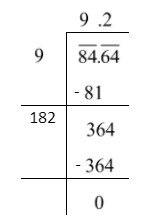QuestionAnswers

# Find the square root of 84.64 using long division method:A. 9.1B. 9.2C. 9.3D. 9.4

Hint: We have to use the long division method over here to find the square root of 84.64. Now since 84.64 has a decimal so we will use the rules corresponding to the long division method for square roots of decimal numbers. In 84.64 the decimal is after 2 places which means the square root will have one digit after the decimal. The digit cannot be 1 or 3 since the second decimal digit in 84.64 does not end with 1 or 9.

Complete step by step solution:
In the problem the number whose square root needs to be evaluated is 84.64 ending in 4 in the second decimal place. So, the digit in the square root of 84.64 will definitely be an even number.
Now, let us start solving for the square root of 84.64 by the long division methodNow, let us understand the long division method we used to find the square root of 84.64 is steps:

Step 1. We will write the number whose square root is to be found and put a bar on the integral part with the digits starting from units placed in pairs. Here it is 84 that is, $\overline {84}$. Similarly for the decimal part we will put the bar on the digits which are grouped in pairs starting with the first digit after the decimal. In this case it will be $\overline {64}$ .

Step 2. For the first two digits we will check and put that digit as the quotient which gives us the nearest whole square to the integral part. In 84.64, the first two digits are 84, so the nearest whole square is that of 9, which is 81, as ${9^2} = 81$. So the first quotient is 9.

Step 3. We will subtract the nearest whole square from the first two digits and put the decimal and bring down the next pair of digits after that. So we will subtract 81 from 84 and get the remainder as 3. Then we will put the decimal after 9 in the quotient and bring down the next pair of digits 64 after the decimal to form the dividend 364.

Step 4. We will take the double of the first quotient obtained in Step 2, and in the units place put the digit so that it becomes the nearest or the exact divisor of the new dividend.
So, for 84.64, the new divisor will now become 18, which is the double of first quotient 9 and the new dividend is 364. So if we put 2 in the unit digit after 18 in the divisor, it will become 182 which will divide 364 exactly twice, leaving no remainder. So we put a 2 in the divisor after 18 and in the quotient also after the decimal, such that the quotient becomes 9.2.
So, 9.2 will be the square root of 84.64.

Hence the correct answer is option B.

Note: We have to be very careful with the pairing of the digits for putting the bar in the first step. If the order in which they are paired is not followed then we will not be able to get the correct square root of the number.